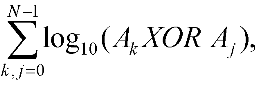ENG  RUS Timus Online JudgeOnline Judge
Problems
Authors
Online contests
Site news
Webboard
Problem set
Submit solution
Judge status
Guide
Register
Authors ranklist
Current contest
Scheduled contests
Past contests
Rules

Contest is over

B. Logarithm

Time limit: 1.0 second
Memory limit: 64 MB
Given a set A of N unordered 128-bit numbers. You are to compute a value of the functionwhere Ak is the kth element of A, log10X — the integer part of the decimal logarithm of X. We’ll assume that log100 = 0.

Input

The first input line contains a number N ≤ 5000. In the following N lines there are 128-bit numbers Ak presented by sets of numbers (a1k, a2k, a3k, a4k), each of them lies in range from 0 to 232-1. The number Ak can be obtained from this set according to the formula
Ak = 296a1k + 264a2k + 232a3k + a4k.

Output

You are to output the value of the function for the given set.

Sample

inputoutput
2
0 0 0 2324
0 2332 0 0
44
Problem Author: Idea: Nikita Shamgunov, prepared by Nikita Shamgunov, Anton Botov
Problem Source: VIII Collegiate Students Urals Programming Contest. Yekaterinburg, March 11-16, 2004
To submit the solution for this problem go to the Problem set: 1318. Logarithm